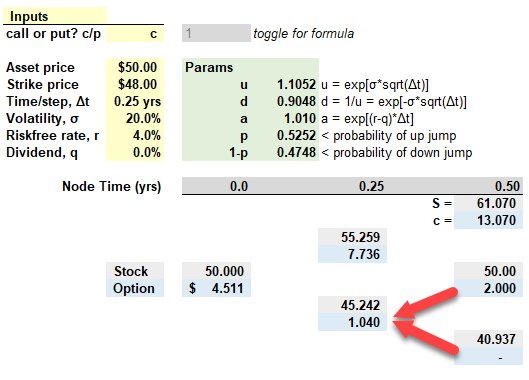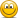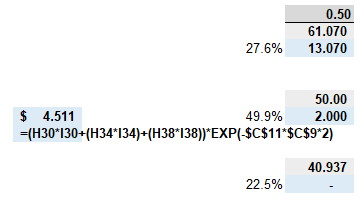# Binomial Tree - Question

#### Eustice_Langham

##### Active Member
Hi, I hope that someone can assist with the below question that is in the GARP Notes:

14.11 A stock price is currently 40. It is known that it will be 42 or 38 at the end of a month. The risk-free rate is 4% per annum with continuous compounding. What is the value of a one-month call option with a strike price of 39?

14.12 In Question 14.11, what position should be taken in the stock to hedge a short position in the option?

I have been able to arrive at the answer for question 14.11, ie 1.595

However the answer for 14.12 is something that I am struggling with, the answer according to the notes is as follows:

"The position is long 0.75 of a share. This is because a portfolio of 0.75 shares and short one option is worth 28.5 for both outcomes"

Could someone explain how this was arrived at? Thanks

#### David Harper CFA FRM

##### David Harper CFA FRM
Staff member
Subscriber
Hi @Eustice_Langham

The option's delta = Δc/ΔS = (3-0)/(42-38) = 0.75. The fundamental delta hedge (i.e., informs the Black-Scholes even) is short one call plus long one Δ share; see my video on this here at https://forum.bionicturtle.com/threads/t4-20-market-makers-delta-hedge-illustrated.22491/

Therefore the hedge is short one call plus long 0.75 shares. At maturity, the payoff is:
• If jump up to S(1/12) = 42.00, portfolio payoff = ΔS - c = 42*0.75 - 3 = $28.50 • If jump down to S(1/12) = 38.00, portfolio payoff = ΔS - c = 38*0.75 - 0 =$28.50
Notice the "perfect hedge" requires that future ΔS(u) - f(u) = ΔS(d) - f(d); i.e., same net payoff regardless of up/down where f(u) and f(d) are option payoffs. Given ΔS(u) - f(u) = ΔS(d) - f(d) --> Δ*[S(u) ΔS(d)] = f(u) - f(d) -->
Δ = [f(u) - f(d)]/[S(u) ΔS(d)]; i.e., delta is the solution to the question, "what fraction of a long share should be hold to equate the payoffs?". I hope that's helpful,

#### Eustice_Langham

##### Active Member
Thanks David, its very useful particularly the video.

May I ask you another question, same source ie GARP, I believe that there looks to be an error in an answer provided to the following question:

14.15 A stock price is currently 50. Its volatility is 20% per annum. The risk-free rate is 4% per annum with continuous compounding. Use a two-step tree to determine the value of a six-month European call option on the stock with a strike price of 48.

The answer provided is 4.511, however the only way that I can see how they have arrived at the number is to include the down leg when calculating the value of the call option.

The value of this leg should be 0 and not 1.040.

u = 1.105
d = .905

prob up = .5252
prob down = .4748

If the down leg stock value is expected at $50*.905 =$45.25, and the strike of the option is $48, then the value of the option should be 0. My estimation is that when calculating the value of the option should be as follows: UU = 13.07 * .5252 * .5252 = 3.61 UD/DU = 2.00 * .5252 * .4748 = .49 = 4.10 4.10 / exp^.04*.5 = 4.02 When including the down leg as the answer provides: D = 1.040 *.4748 = .49 UU = 13.07 * .5252 * .5252 = 3.61 UD/DU = 2.00 * .5252 * .4748 = .49 =4.59/exp^.04*.5 = 4.51 Thanks #### David Harper CFA FRM ##### David Harper CFA FRM Staff member Subscriber Hi @Eustice_Langham I agree with GARP's answer. I think your mistake it to treat the call option value as zero at node c[date 1, state 0] where the stock price is 45.242. You are correct that with a strike price of 48.00, the option would be underwater at node[1,0] but we only use intrinsic value at the terminal node. At this node c(1,0), this European option still has three months of remaining time value: it must have a value greater than zero! The value of all other (i.e., non-terminal) nodes are their expected discounted value (DEV); so the value of node(1,0) = ($2.00 * 0.5252 + $0 * 0.4748)*exp(-4%*0.25) =$1.040. You can view this as node(1,0) = DEV = expected_value*discount_factor. See image below. My XLS is here https://www.dropbox.com/s/nwszy3ozl6nwoja/080820-garp-eoc-15-15.xlsx?dl=0
... please note this is a European call. If this were an American call, the value of each non-terminal node is given by max(DEV, intrinsic value) to reflect that the holder of an American option could early exercise. I hope that's helpful!#### Eustice_Langham

##### Active Member
Thanks David again, I wasn't expecting a thorough response.

One more question:

1. To arrive at the option value $4.511, in your spreadsheet, you have only included the values at the 0.25 time step only, and not the$13.070 value, is this because of backward induction ie valuing the option gong back through the tree?

#### David Harper CFA FRM

##### David Harper CFA FRM
Staff member
Subscriber
Hi @Eustice_Langham You put effort into your question, so I think (maybe even if somewhat subconsciously) I tend to requite such sincerity with a bit more effort on my endYes, it is exactly backward induction. To honor the flexibility of the model, we normally discount each interval successively. But please see below (and I added this to the same XLS). Under these clean European assumptions (i.e., nodes are not MAX functions), we get the exact same result by discounting the option values from maturity. In this case, $4.511 = (($13.070 * p^2) + [$2.00 * p*(1-p)*2] + [$0 * (1-p)^2])*exp(-4.0%*0.25*2), where p^2 = 27.6% and p*(1-p)*2 = 49.9%. I have to be careful that the probabilities are indeed a complete binomial distribution; i.e., every column in the tree is a complete binomial distribution. So the p[date 2, 1] = p*(1-p)*2 not p*(1-p) and the sum of 27.6% + 49.9% + 22.5% = 100.0%. In which case, you'll see that (also) the discount factor is exp(-4.0%*0.25*2) = exp(-4.0%*0.50). But this value of \$4.511 is exactly the same, it's not even an approximation by virtue of the math. Thanks!#### Eustice_Langham

##### Active Member
Thanks again David, its clear now. I have been using the spreadsheet for American style options as well to corroborate my own attempts and it works, so the penny has successfully dropped. Thanks